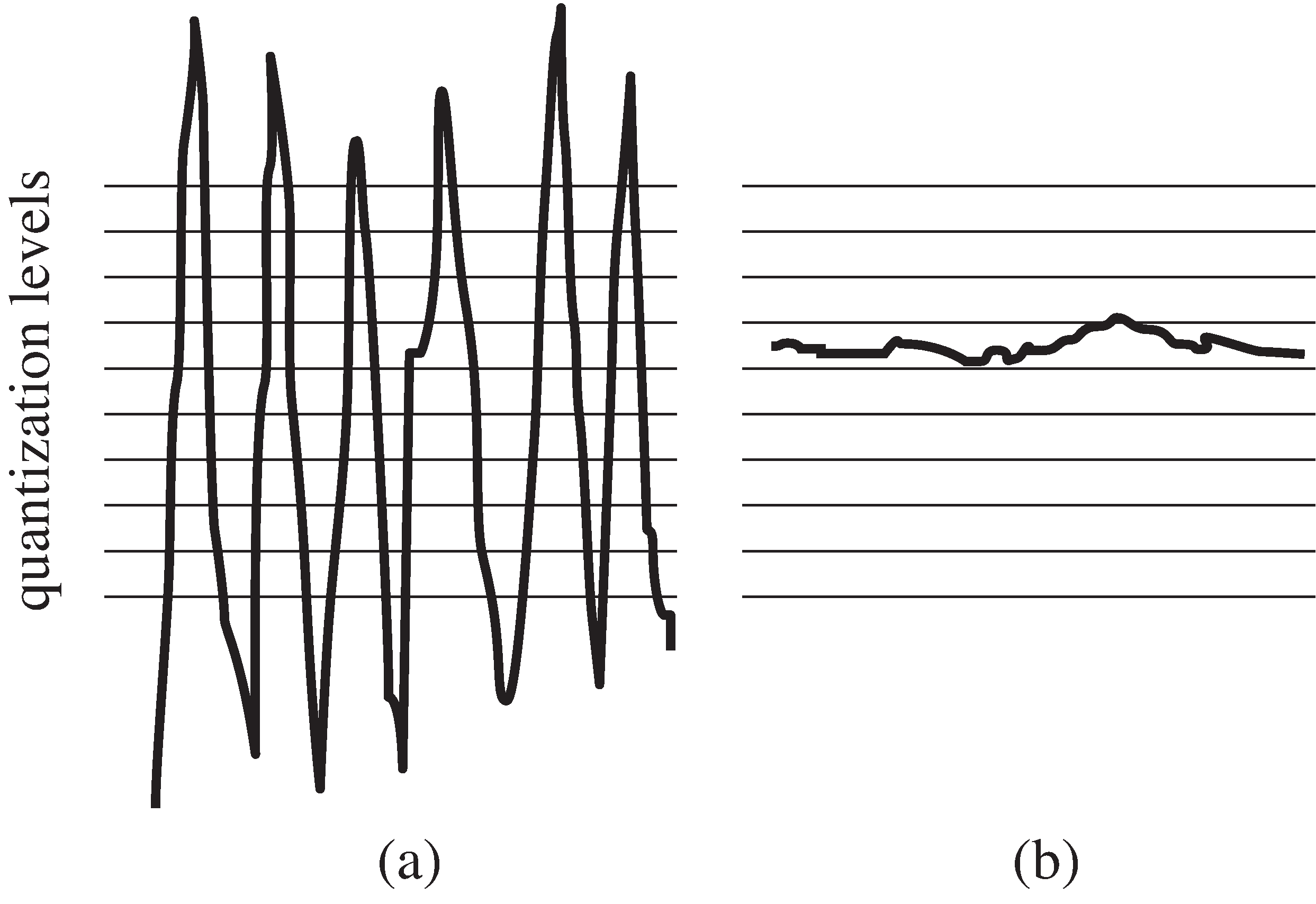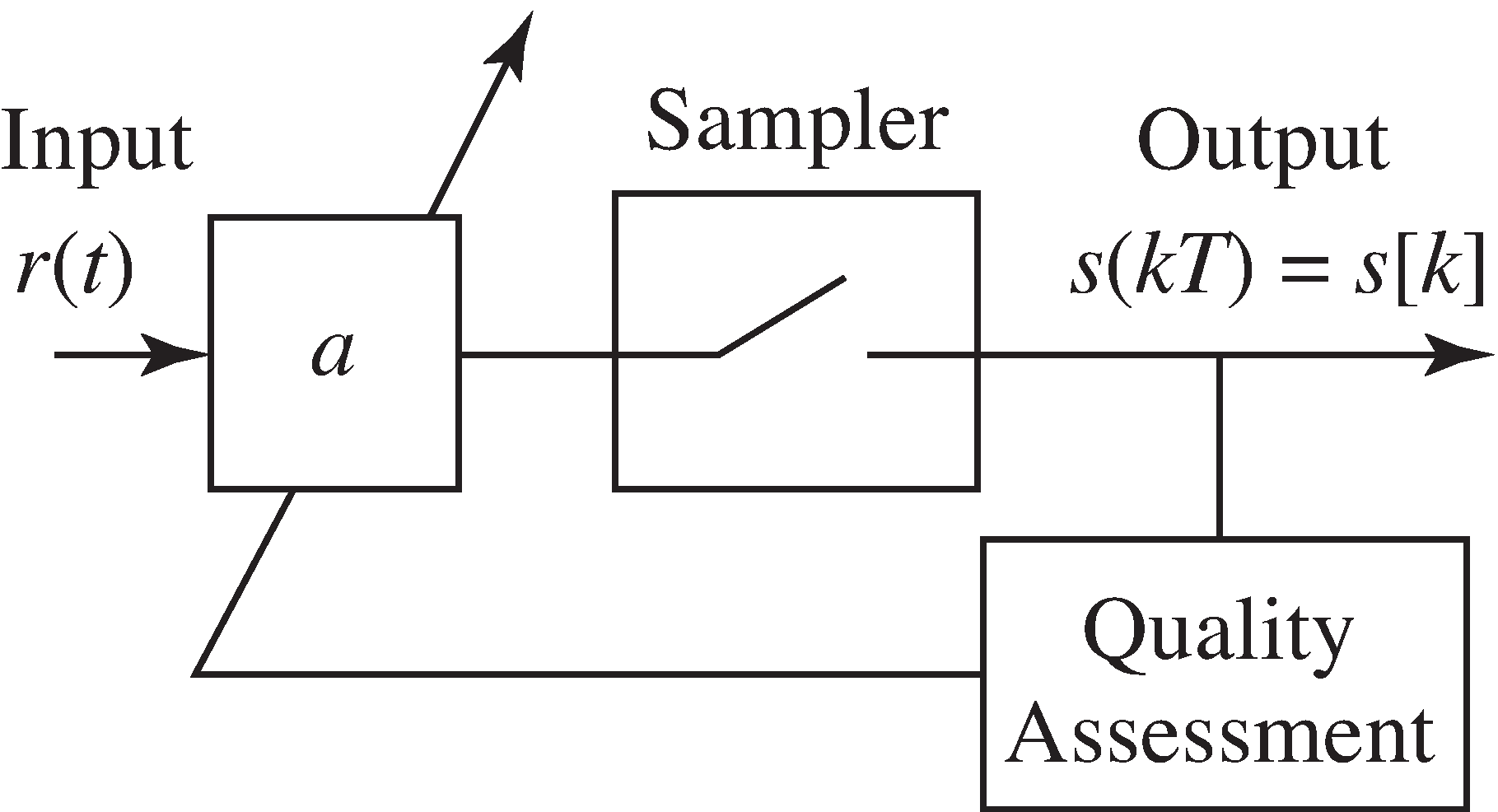0.5 Sampling with automatic gain control  (Page 15/19)

 Page 15 / 19

There are two basic approaches to an AGC. The traditional approach uses analog circuitry to adjust the gain before the sampling. The more modern approach uses the output of the sampler to adjust thegain. The advantage of the analog method is that the two blocks (the gain and the sampling) are separate and do not interact.The advantage of the digital adjustment is that less additional hardware is required since the DSP processing is already presentfor other tasks.The goal of the AGC is to maintain the dynamic range of the signal by attenuating it when it is too large (as in (a))and by increasing it when it is too small (as in (b)).

A simple digital system for AGC gain adjustment is shown in [link] . The input $r\left(t\right)$ is multiplied by the gain $a$ to give the normalized signal $s\left(t\right)$ . This is then sampled to give the output $s\left[k\right]$ . The assessment block measures $s\left[k\right]$ and determines whether $a$ must be increased or decreased.An automatic gain control adjusts the gain parameter a so that the average energy at the output remains (roughly) fixed, despitefluctuations in the average received energy.

The goal is to choose $a$ so that the power (or average energy) of $s\left(t\right)$ is approximately equal to some specified ${\mathbf{s}}^{2}$ . Since

${a}^{2}{\left(\text{avg},\left\{,{r}^{2},\left(t\right),\right\}|}_{t=kT}\approx \text{avg}\left\{{s}^{2}\left(kT\right)\right\}\approx \text{avg}\left\{{s}^{2}\left[k\right]\right\},$

it would be ideal to choose

${a}^{2}\approx \frac{{\mathbf{s}}^{2}}{\text{avg}\left\{{r}^{2}\left(kT\right)\right\}},$

because this would imply that $\text{avg}\left\{{s}^{2}\left(kT\right)\right\}\approx {\mathbf{s}}^{2}$ . The averaging operation (in this case a movingaverage over a block of data of size $N$ ) is defined by

$\text{avg}\left\{x\left[k\right]\right\}=\frac{1}{N}\sum _{i=k-N+1}^{k}x\left[i\right]$

Unfortunately, neither the analog input $r\left(t\right)$ nor its power are directly available to the assessment blockin the DSP portion of the receiver, so it is not possible to directly implement [link] .

Is there an adaptive element that can accomplish this task? As suggested in the beginning of "Iteration and Optimization" , there are three steps to the creation of a viableoptimization approach: setting a goal, choosing a solution method, and testing. As in any real life engineering task, a propermathematical statement of the goal can be tricky, and this section proposes two (slightly different) possibilitiesfor the AGC. By comparing the resulting algorithms (essentially, alternativeforms for the AGC design), it may be possible to trade off among various design considerations.

One sensible goal is to try to minimize a simple function of the difference between the power of the sampled signal $s\left[k\right]$ and the desired power ${\mathbf{s}}^{2}$ . For instance, the averaged squared error in the powers of $s$ and $\mathbf{s}$ ,

$\begin{array}{cc}\hfill {J}_{LS}\left(a\right)& =\text{avg}\left\{\frac{1}{4},{\left({s}^{2}\left[k\right]-{\mathbf{s}}^{2}\right)}^{2}\right\}\hfill \\ & =\frac{1}{4}\text{avg}\left\{{\left({a}^{2}{r}^{2}\left(kT\right)-{\mathbf{s}}^{2}\right)}^{2}\right\},\hfill \end{array}$

penalizes values of $a$ which cause ${s}^{2}\left[k\right]$ to deviate from ${\mathbf{s}}^{2}$ . This formally mimics the parabolic form of the objective [link] in the polynomial minimization example of the previous section.Applying the steepest descent strategy yields

$a\left[k+1\right]=a\left[k\right]-\mu {\left(\frac{d{J}_{LS}\left(a\right)}{da}|}_{a=a\left[k\right]},$

which is the same as [link] , except that the name of the parameter has changed from $x$ to $a$ . To find the exact form of [link] requires the derivative of ${J}_{LS}\left(a\right)$ with respect to the unknown parameter $a$ . This can be approximated by swapping the derivative and the averaging operations to give

$\begin{array}{c}\\ \hfill \frac{d{J}_{LS}\left(a\right)}{da}& =& \frac{1}{4}\frac{d\text{avg}\left\{{\left({a}^{2}{r}^{2}\left(kT\right)-{\mathbf{s}}^{2}\right)}^{2}\right\}}{da}\hfill \\ & \approx & \frac{1}{4}\text{avg}\left\{\frac{d{\left({a}^{2}{r}^{2}\left(kT\right)-{\mathbf{s}}^{2}\right)}^{2}}{da}\right\}\hfill \\ & =& \text{avg}\left\{\left({a}^{2}{r}^{2}\left(kT\right)-{\mathbf{s}}^{2}\right)a{r}^{2}\left(kT\right)\right\}.\hfill \end{array}$

what is math number
x-2y+3z=-3 2x-y+z=7 -x+3y-z=6
Need help solving this problem (2/7)^-2
x+2y-z=7
Sidiki
what is the coefficient of -4×
-1
Shedrak
the operation * is x * y =x + y/ 1+(x × y) show if the operation is commutative if x × y is not equal to -1
An investment account was opened with an initial deposit of $9,600 and earns 7.4% interest, compounded continuously. How much will the account be worth after 15 years? Kala Reply lim x to infinity e^1-e^-1/log(1+x) given eccentricity and a point find the equiation Moses Reply 12, 17, 22.... 25th term Alexandra Reply 12, 17, 22.... 25th term Akash College algebra is really hard? Shirleen Reply Absolutely, for me. My problems with math started in First grade...involving a nun Sister Anastasia, bad vision, talking & getting expelled from Catholic school. When it comes to math I just can't focus and all I can hear is our family silverware banging and clanging on the pink Formica table. Carole I'm 13 and I understand it great AJ I am 1 year old but I can do it! 1+1=2 proof very hard for me though. Atone hi Adu Not really they are just easy concepts which can be understood if you have great basics. I am 14 I understood them easily. Vedant find the 15th term of the geometric sequince whose first is 18 and last term of 387 Jerwin Reply I know this work salma The given of f(x=x-2. then what is the value of this f(3) 5f(x+1) virgelyn Reply hmm well what is the answer Abhi If f(x) = x-2 then, f(3) when 5f(x+1) 5((3-2)+1) 5(1+1) 5(2) 10 Augustine how do they get the third part x = (32)5/4 kinnecy Reply make 5/4 into a mixed number, make that a decimal, and then multiply 32 by the decimal 5/4 turns out to be AJ how Sheref can someone help me with some logarithmic and exponential equations. Jeffrey Reply sure. what is your question? ninjadapaul 20/(×-6^2) Salomon okay, so you have 6 raised to the power of 2. what is that part of your answer ninjadapaul I don't understand what the A with approx sign and the boxed x mean ninjadapaul it think it's written 20/(X-6)^2 so it's 20 divided by X-6 squared Salomon I'm not sure why it wrote it the other way Salomon I got X =-6 Salomon ok. so take the square root of both sides, now you have plus or minus the square root of 20= x-6 ninjadapaul oops. ignore that. ninjadapaul so you not have an equal sign anywhere in the original equation? ninjadapaul hmm Abhi is it a question of log Abhi 🤔. Abhi I rally confuse this number And equations too I need exactly help salma But this is not salma it's Faiza live in lousvile Ky I garbage this so I am going collage with JCTC that the of the collage thank you my friends salma Commplementary angles Idrissa Reply hello Sherica im all ears I need to learn Sherica right! what he said ⤴⤴⤴ Tamia hii Uday hi salma hi Ayuba Hello opoku hi Ali greetings from Iran Ali salut. from Algeria Bach hi Nharnhar A soccer field is a rectangle 130 meters wide and 110 meters long. The coach asks players to run from one corner to the other corner diagonally across. What is that distance, to the nearest tenths place. Kimberly Reply Jeannette has$5 and \$10 bills in her wallet. The number of fives is three more than six times the number of tens. Let t represent the number of tens. Write an expression for the number of fives.
What is the expressiin for seven less than four times the number of nickels
How do i figure this problem out.
how do you translate this in Algebraic Expressions
why surface tension is zero at critical temperature
Shanjida
I think if critical temperature denote high temperature then a liquid stats boils that time the water stats to evaporate so some moles of h2o to up and due to high temp the bonding break they have low density so it can be a reason
s.
Need to simplify the expresin. 3/7 (x+y)-1/7 (x-1)=
. After 3 months on a diet, Lisa had lost 12% of her original weight. She lost 21 pounds. What was Lisa's original weight?
Got questions? Join the online conversation and get instant answers!ByBy Jordon HumphreysByByByBy